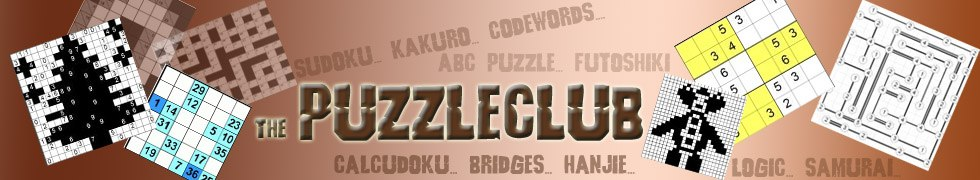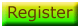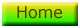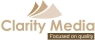# ABC Puzzle Answer

Here is the answer to the sample ABC Puzzle. We have used 'x' as a symbol to denote the two empty cells in each row or column, if you haven't marked them at all or have used a different symbol then that's absolutely fine!

If you enjoyed this puzzle, then why not play many more ABC Puzzles within the Puzzle Club!

 B C C B X X A A X A C B X B X X B A C C B X A C X C A A C X X B C C©   |     |